FLAC3D Theory and Background • Constitutive Models

# Borehole Behavior in Elastic Anisotropic Materials (FLAC3D)

Note

To view this project in FLAC3D, use the menu command Help ► Examples…. Choose “ConstitutiveModels/ BoreholeAnisotropicElastic” and select “BoreholeAnisotropicElastic.prj” to load. The project’s main data file is shown at the end of this example.

The plane-stress problem of a circular tunnel in an elastic, transversely isotropic medium subjected to an isotropic stress field at infinity is considered for this verification test. The solution is compared to an analytic solution for radial and tangential displacement, and for tangential stress given by Amadei (1982).

The radius of the tunnel is 1 m. The isotropic in-situ stress is 10 MPa. Due to symmetry, only a quarter domain is considered. The grid is as Figure 1.

The benchmark material is anisotropic elastic material, but the validation is not limited to the anisotropic elastic material. Another three more complex models with material properties degraded to the equivalent properties of the anisotropic elastic model are tested as well.

Anisotropic Model

For anisotropic elastic model, the material properties are listed in Table 1. (see the datafile borehole-aniso.dat below).

 $$E_1$$ $$E_3$$ $$G_{13}$$ $$\nu_1$$ $$\nu_3$$ dip dip-direction 40e3 20e3 4e3 0.2 0.1 0 0

The numerical results are compared with analytic solutions as followed: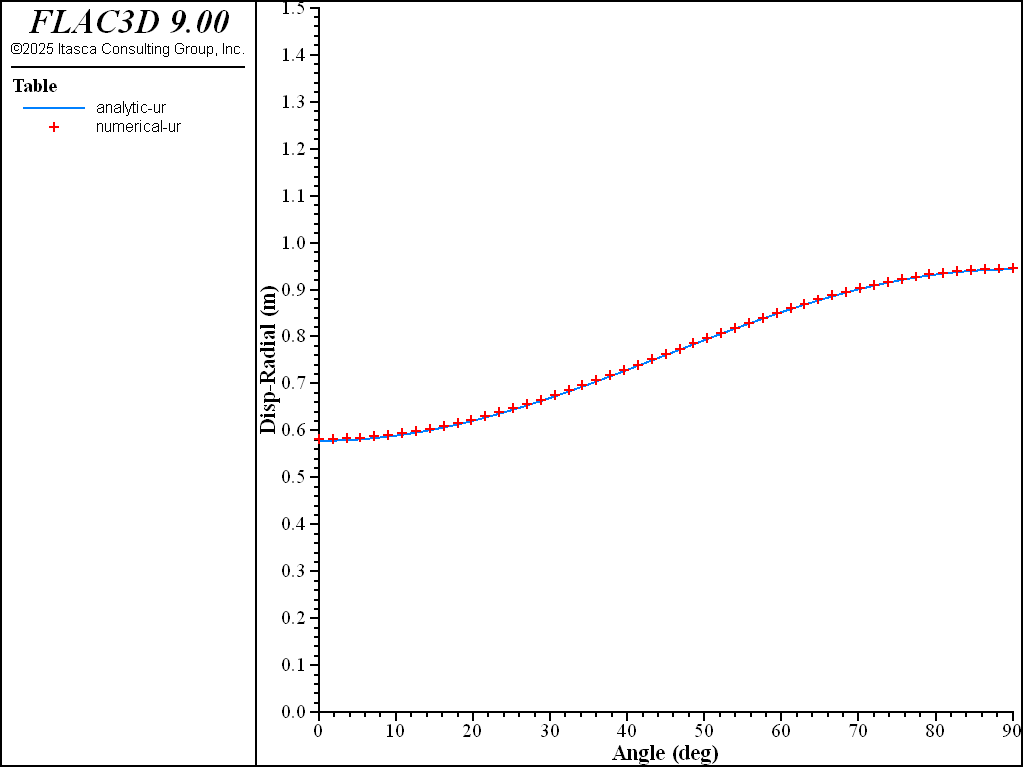Figure 2: Surface radial displacement versus angle $$\theta$$ — comparison between numerical and analytical solutions.Figure 3: Surface tangential displacement versus angle $$\theta$$ — comparison between numerical and analytical solutions.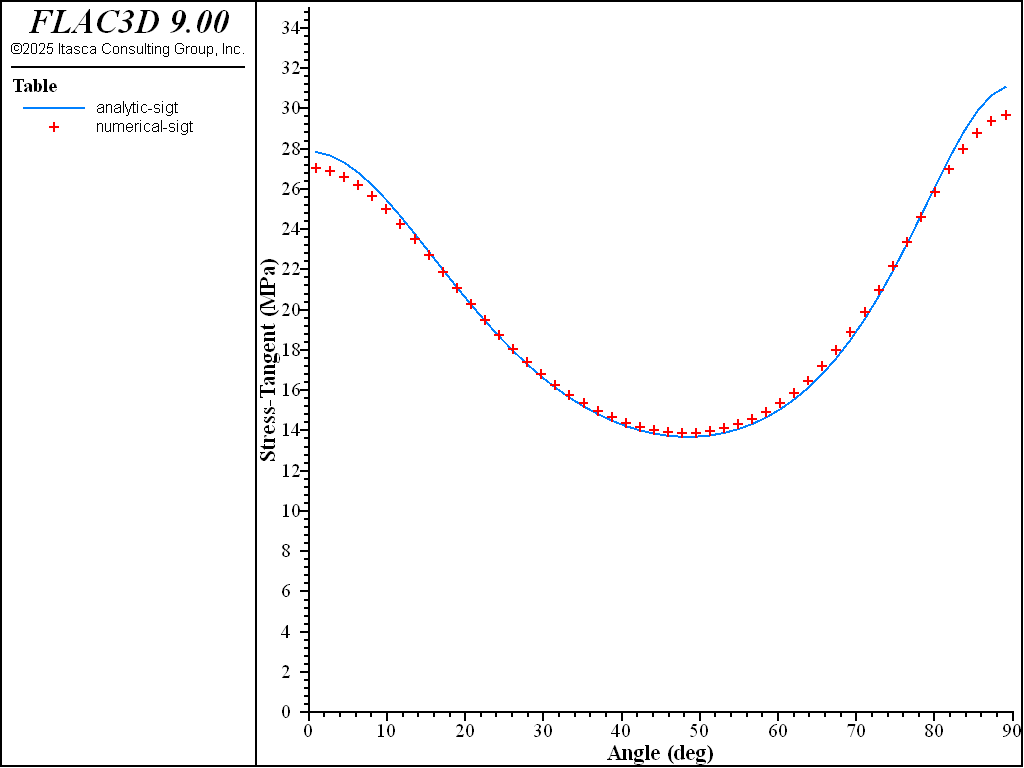Figure 4: Near-surface tangential stress versus angle $$\theta$$ — comparison between numerical and analytical solutions.

Orthotropic Model

For orthotropic elastic model, the material properties are listed in Table 2. (see the datafile borehole-ortho.dat below).

 $$E_{1}$$ ($$E_{2}$$) $$E_3$$ $$G_{13}$$ ($$G_{23}$$) $$\nu_{12}$$ $$\nu_{13}$$ ($$\nu_{23}$$) $$G_{12}$$ dip dip-direction 40e3 20e3 4e3 0.2 0.1 16.7e3 0 0

The numerical results are compared with analytic solutions as followed:Figure 5: Surface radial displacement versus angle $$\theta$$ — comparison between numerical and analytical solutions.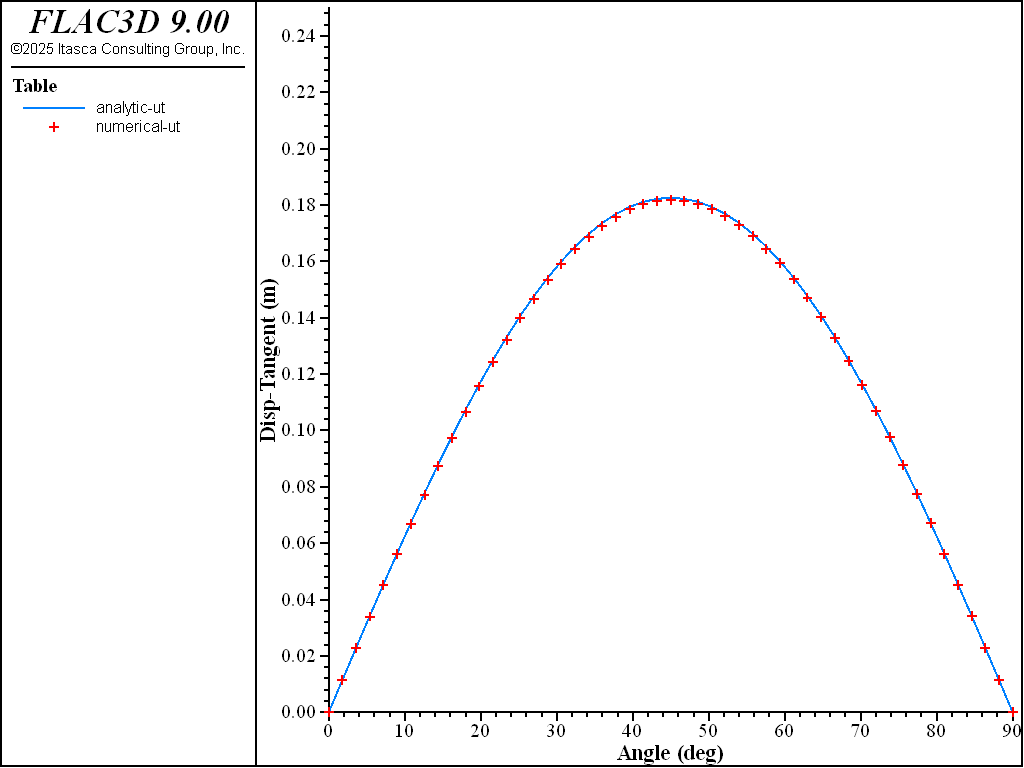Figure 6: Surface tangential displacement versus angle $$\theta$$ — comparison between numerical and analytical solutions.Figure 7: Near-surface tangential stress versus angle $$\theta$$ — comparison between numerical and analytical solutions.

Ubiquitous-Anisotropic Model

For ubiquitous-anisotropic elastic model, the material properties are the same as Table 1, but the strength properties of the joints are set to high values to allow the model to behave elastically. (see the datafile borehole-caniso.dat below).

The numerical results are compared with analytic solutions as followed:Figure 8: Surface radial displacement versus angle $$\theta$$ — comparison between numerical and analytical solutions.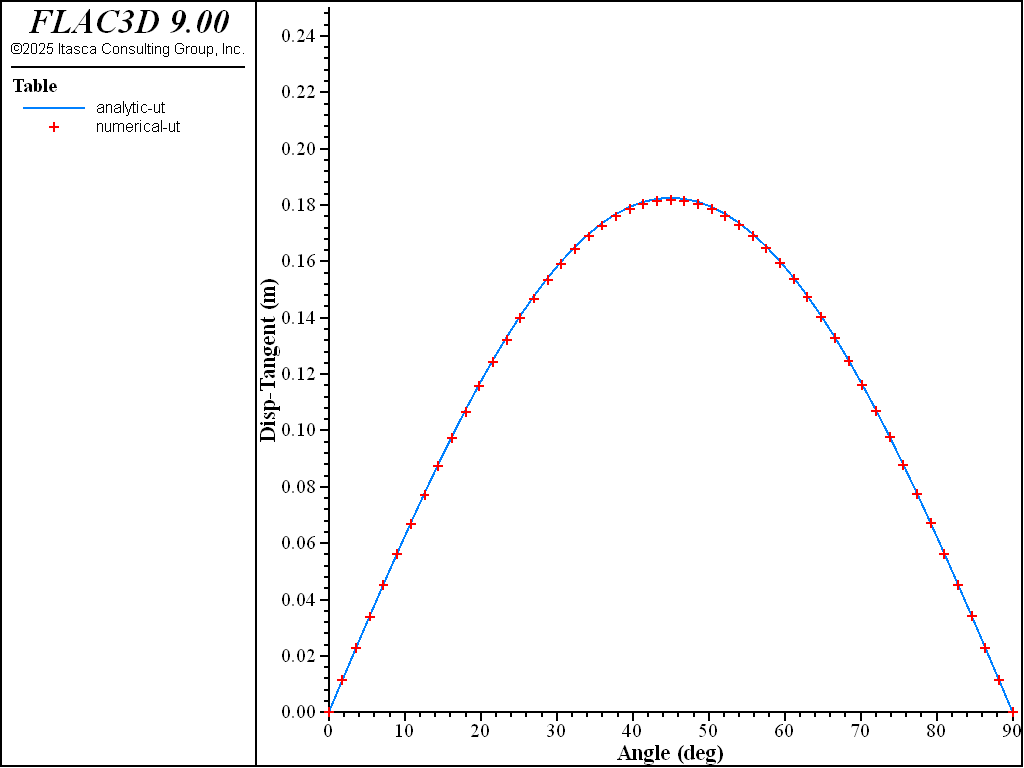Figure 9: Surface tangential displacement versus angle $$\theta$$ — comparison between numerical and analytical solutions.Figure 10: Near-surface tangential stress versus angle $$\theta$$ — comparison between numerical and analytical solutions.

Columnar-Basalt (COMBA) model

For columnar-basalt (COMBA) model, three orthogonal ubiquitous planes are selected. The plane of isotropy (ubiquitous joint 3) is normal to the z-axis. Plane 2 is normal to the x-axis, and plane 1 is normal to the y-axis. The strength properties of the joints are set to high values to allow the model to behave elastically. The joint spacing is 10 cm for the ubiquitous joints. (see the datafile borehole-comba.dat below).

The numerical results are compared with analytic solutions as followed: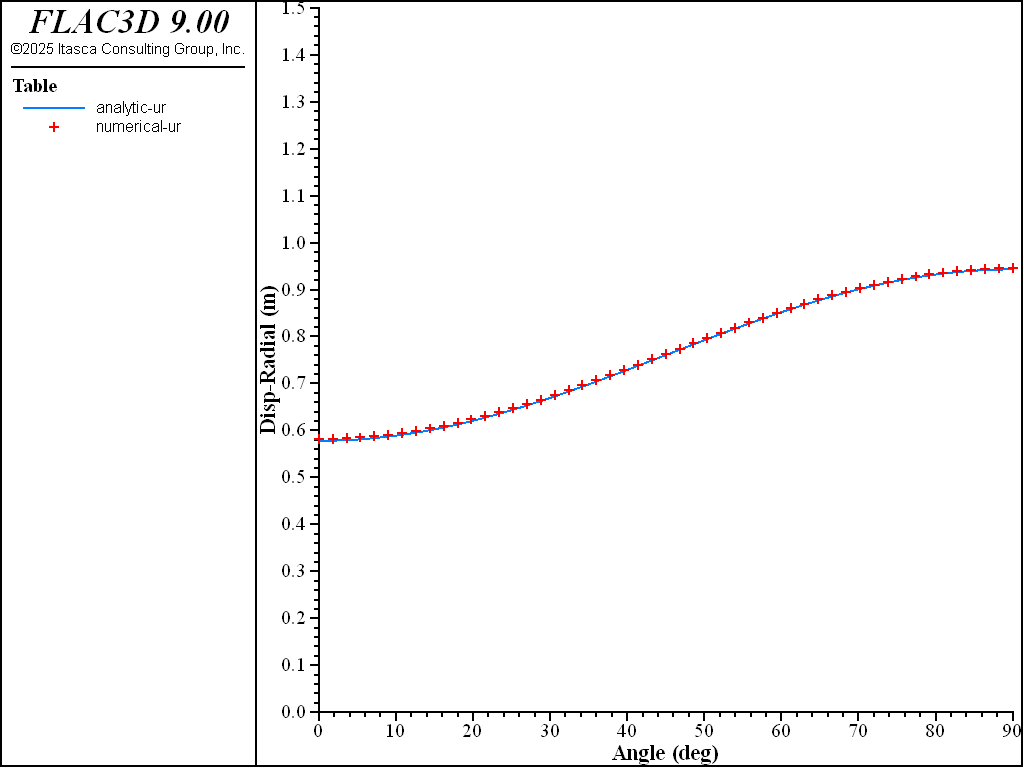Figure 11: Surface radial displacement versus angle $$\theta$$ — comparison between numerical and analytical solutions.Figure 12: Surface tangential displacement versus angle $$\theta$$ — comparison between numerical and analytical solutions.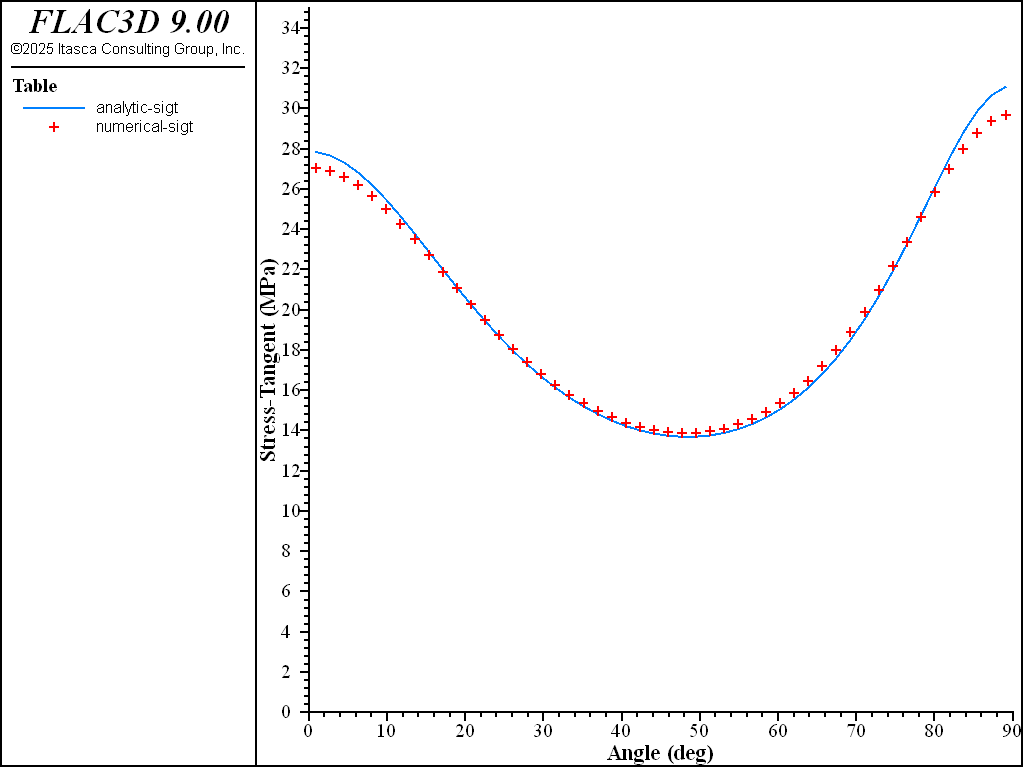Figure 13: Near-surface tangential stress versus angle $$\theta$$ — comparison between numerical and analytical solutions.

References

Amadei, B. “The Influence of Rock Anisotropy on Measurement of Stresses In-Situ.” Ph.D. Thesis, University of California, Berkeley (1982).

Data File

borehole-aniso.dat

;------------------------------------------------------------------
;  Numerical solution for a long tunnel in pre-stressed
;  anisotropic material - plane stress
;------------------------------------------------------------------
model new
model large-strain off
zone create radial-cylinder size 1 1 50 50 ratio 1 1 1 1.1 ...
point 1 20 0 0 point 2 0 0.2 0 point 3 0 0 20 dimension 1 1 1 1

zone cmodel assign anisotropic
zone property young-plane 40e3 young-normal 20e3 shear-normal 4e3
zone property poisson-plane 0.2 poisson-normal 0.1
zone property dip 0 dip-direction 0
;
zone initialize stress-xx -10
zone initialize stress-zz -10
zone gridpoint fix velocity-z range position-z 0
zone gridpoint fix velocity-x range position-x 0
zone face apply stress-xx -10 range position-x 20
zone face apply stress-zz -10 range position-z 20
;
zone history displacement-x position 1 0 0
zone history velocity-x position 1 0 0
history interval 20
model solve convergence 1

program call "solu"
model save 'borehole-aniso'


borehole-ortho.dat

;------------------------------------------------------------------
;  Numerical solution for a long tunnel in pre-stressed
;  anisotropic material - plane stress
;------------------------------------------------------------------
model new
model large-strain off
zone create radial-cylinder size 1 1 50 50 ratio 1 1 1 1.1 ...
point 1 20 0 0 point 2 0 0.2 0 point 3 0 0 20 dimension 1 1 1 1

zone cmodel assign orthotropic
zone property young-1 40e3 young-2 40e3 young-3 20e3
zone property poisson-12 0.2 poisson-13 0.1 poisson-23 0.1
zone property shear-13 4e3 shear-23 4e3 shear-12 16.67e3
zone property dip 0 dip-direction 0
;
zone initialize stress-xx -10
zone initialize stress-zz -10
zone gridpoint fix velocity-z range position-z 0
zone gridpoint fix velocity-x range position-x 0
zone face apply stress-xx -10 range position-x 20
zone face apply stress-zz -10 range position-z 20
;
zone history displacement-x position 1 0 0
zone history velocity-x position 1 0 0
history interval 20
model solve convergence 1

program call "solu"
model save 'borehole-ortho'


borehole-caniso.dat

;------------------------------------------------------------------
;  Numerical solution for a long tunnel in pre-stressed
;  anisotropic material - plane stress
;------------------------------------------------------------------
model new
model large-strain off
zone create radial-cylinder size 1 1 50 50 ratio 1 1 1 1.1 ...
point 1 20 0 0 point 2 0 0.2 0 point 3 0 0 20 dimension 1 1 1 1

zone cmodel assign ubiquitous-anisotropic
zone property young-plane 40e3 young-normal 20e3 shear-normal 4e3
zone property poisson-plane 0.2 poisson-normal 0.1
zone property dip 0 dip-direction 0
zone property cohesion 1e20 tension 1e20
zone property joint-cohesion 1e20 joint-tension 1e20
;
zone initialize stress-xx -10
zone initialize stress-zz -10
zone gridpoint fix velocity-z range position-z 0
zone gridpoint fix velocity-x range position-x 0
zone face apply stress-xx -10 range position-x 20
zone face apply stress-zz -10 range position-z 20
;
zone history displacement-x position 1 0 0
zone history velocity-x position 1 0 0
history interval 20
model solve convergence 1

program call "solu"
model save 'borehole-caniso'


borehole-comba.dat

;------------------------------------------------------------------
;  Numerical solution for a long tunnel in pre-stressed
;  anisotropic material - plane stress
;------------------------------------------------------------------
model new
model large-strain off
zone create radial-cylinder size 1 1 50 50 ratio 1 1 1 1.1 ...
point 1 20 0 0 point 2 0 0.2 0 point 3 0 0 20 dimension 1 1 1 1

zone cmodel assign columnar-basalt
zone property young=60e3 poisson=0.30
zone property space-1=0.1 space-2=0.1 space-3=0.1
zone property stiff-normal-1=120e4 stiff-normal-2=120e4 stiff-normal-3=30e4
zone property stiff-shear-1=120e4 stiff-shear-2=120e4 stiff-shear-3=5.04e4
zone property normal-x-1 0 normal-y-1 1 normal-z-1 0
zone property normal-x-2 1 normal-y-2 0 normal-z-2 0
zone property normal-x-3 0 normal-y-3 0 normal-z-3 1
zone property j-cohesion-1 1e20 j-tension-1 1e20
zone property j-cohesion-2 1e20 j-tension-2 1e20
zone property j-cohesion-3 1e20 j-tension-3 1e20
zone property j-cohesion-4 1e20 j-tension-4 1e20
;
zone initialize stress-xx -10
zone initialize stress-zz -10
zone gridpoint fix velocity-z range position-z 0
zone gridpoint fix velocity-x range position-x 0
zone face apply stress-xx -10 range position-x 20
zone face apply stress-zz -10 range position-z 20
;
zone history displacement-x position 1 0 0
zone history velocity-x position 1 0 0
history interval 20
model solve convergence 1

program call "solu"
model save 'borehole-comba'